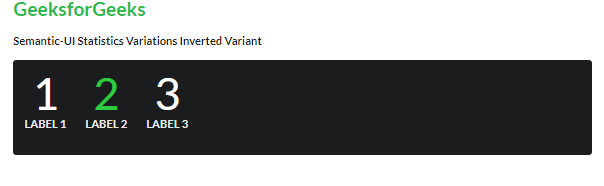Open in App
Not now

# Semantic-UI Statistics Variations Inverted Variant

• Last Updated : 12 Feb, 2022

Semantic UI is an open-source framework that offers a variety of components to make your UI more meaningful. One of them is “Statistics”.

Semantic UI has a Statistics component to magnify particular data for your website. Statistics values can be shown in different colors as per requirement. Users can also display the statistics in a dark background. Let us see this variation of statistics.

If you want to display statistics on black background users can use inverted statistics. A single or group of statistics showing relatable content can be displayed in dark background. We will create a UI to show the statistics inverted variation.

Semantic-UI Statistics Inverted Variation Class:

• inverted: This class is used to make the statistics inverted(color-wise).

Syntax:

```<div class="ui inverted segment">
<div class="ui inverted statistic">
.....
</div>
</div>```

Example 1: This example demonstrates statistics inverted variation by using inverted class.

## HTML

 ` ` `<``html``> ` ` `  `<``head``> ` `   ``<``link` `href``= ` `"https://cdnjs.cloudflare.com/ajax/libs/semantic-ui/2.4.1/semantic.min.css"`  `         ``rel``=``"stylesheet"` `/> ` ` ` ` `  `<``body``> ` `   ``<``div` `class``=``"ui container"``> ` `      ``<``h2` `class``=``"ui header green"``>GeeksforGeeks ` `      ``<``b``> ` `        ``Semantic-UI Statistics Variations Inverted Variant ` `      `` ` `      ``<``div` `class``=``"ui inverted segment"``> ` `         ``<``div` `class``=``"ui  inverted  statistic"``> ` `            ``<``div` `class``=``"value"``> ` `               ``1 ` `            `` ` `            ``<``div` `class``=``"label"``> ` `               ``Label 1 ` `            `` ` `         `` ` `         ``<``div` `class``=``"ui green inverted statistic"``> ` `            ``<``div` `class``=``"value"``> ` `               ``2 ` `            `` ` `            ``<``div` `class``=``"label"``> ` `               ``Label 2 ` `            `` ` `         `` ` `         ``<``div` `class``=``"ui  inverted statistic"``> ` `            ``<``div` `class``=``"value"``> ` `               ``3 ` `            `` ` `            ``<``div` `class``=``"label"``> ` `               ``Label 3 ` `            `` ` `         `` ` `      `` ` `   `` ` ` ` ` `  ``

Output: You can see in the first row all the statistics in the dark background. All the values of the statistics are in the dark background since the statistics are tagged with inverted class.  It can be easily interpreted through the code that dark background statistics with white color are visible as shown in the figure by adding the inverted class.Semantic-UI Statistics Variations Inverted Variant

My Personal Notes arrow_drop_up
Related Articles